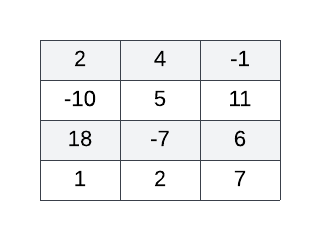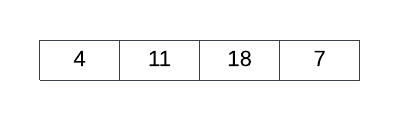Login or Create an Account to view question mark schemes, comment, and download a PDF of this test
Question 1[5 Marks]
Given a 2D array called ARR of size 4x3 and an empty 1D array called BIGGEST of size 4, construct an algorithm to sequentially fill BIGGEST with the biggest number in each ARR row such that the index of each number in BIGGEST is the same as its original ARR row index. The more positive or less negative a number, the bigger it is - for example, 7, 0 and -2 are all considered bigger than -10. ARR may contain one or more negative numbers. For example, consider the following 2D array:The correctly functioning algorithm applied to the 2D array above yields the following 1D array:Question 2[7 Marks]
Construct pseudocode to populate a 1D array of size 100 called FILTERED with as much data from a collection called NUMBERS as will fit and not attempt to write outside the bounds of the array. Fill FILTERED sequentially starting from position . Include in FILTERED only those numbers from the collection NUMBERS which are divisible by 7.

HomeThe Right Test - Fast